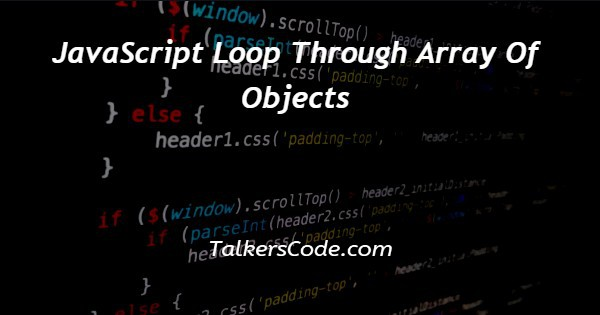# JavaScript Loop Through Array Of Objects

Last Updated : Jan 1, 2023In this tutorial we will show you the solution of JavaScript loop through array of objects, there are many ways to help and we can loop through array of object using JavaScript.

For a better understanding, let us see the example given below.

## Step By Step Guide On JavaScript Loop Through Array Of Objects :-

The ways with help of which we can loop through an array of object using JavaScript are:

• With the help of foreach() function
• With the help of for…of method
• Object. entries method
• Object.values method

In this tutorial, we are going to understand our first way which is with the help of foreach() function.

So, let us see the codes given below to understand how to use foreach() function inside the codes.

```<script>
let details = [
{
name:"robert"
},
{
name:"steve"
},
{
name:"tom"
}
]
details.forEach((data)=>console.log(data.name));
const laptop = [
{
brand: 'HP',
reviews: 'BEST'
},
{
brand: 'DELL',
reviews: 'BEST'
},
{
brand: 'MICROSOFT',
reviews: 'BETTER'
}
];
laptop.forEach(data => {
for (let key in data) {
console.log(`\${key}: \${data[key]}`);
}
});
</script>```
1. As we here see that we today write only JavaScript codes in our example.
2. In previous tutorials, we already many times create a full code example in which we first create an HTML form with its basic structure. Inside that structure, we use HTML, head, title, body, and script tags.
3. In this example, as we see only, we write our JavaScript codes. And inside these codes, we see that how to loop through an object in JavaScript. We hope you know about what is the difference between objects and arrays. If you did not know the difference, then you can visit our article in which we show you what is the difference between an array of objects and an array.
4. Now, in this article as we know that we are going to discuss how to loop through array of object using JavaScript. So, for this we give you two examples. Let us understand both of them. In first, we create an array of object having name inside this. After this to use loop inside this we get help of foreach loop and use in on array and just console the required result. So, this using this we get the data that we stored inside the name.
5. After this in second example. We use two values inside of one in array and create objects inside array. Now, we using the loop we are going to access all the element and show the result. So, for this we first use for loop and after this we use for…in loop to get the values and keys respectively and inside this example when you see the result, we see that we get values as well as keys also.
6. So, with the help of this article we understand the two methods to use foreach loop to loop through an array of object.

## Conclusion :-

At last, in conclusion, here we can say that with the help of this article we can understand how to loop through an object using JavaScript.

I hope this tutorial on JavaScript loop through array of objects helps you and the steps and method mentioned above are easy to follow and implement.